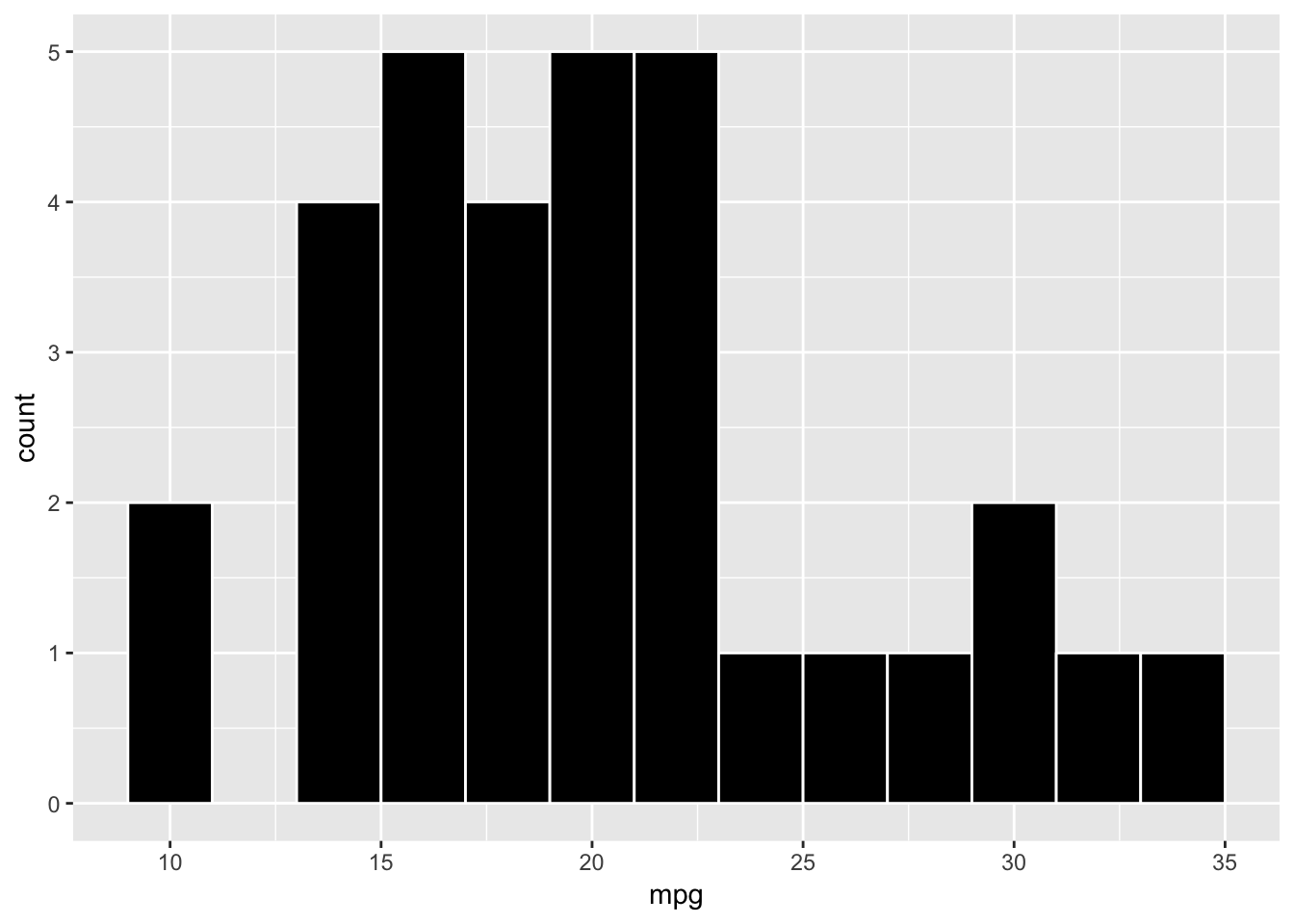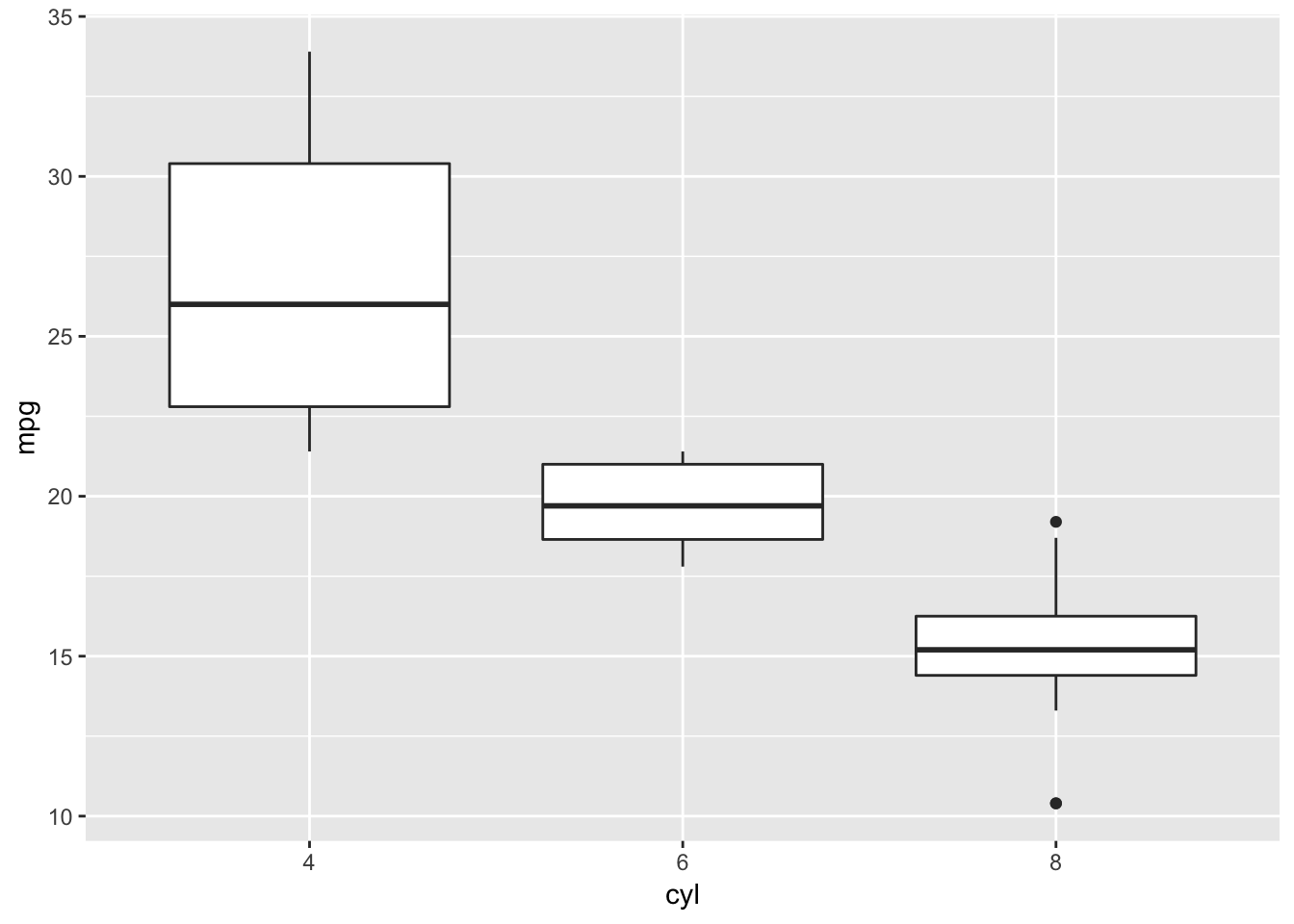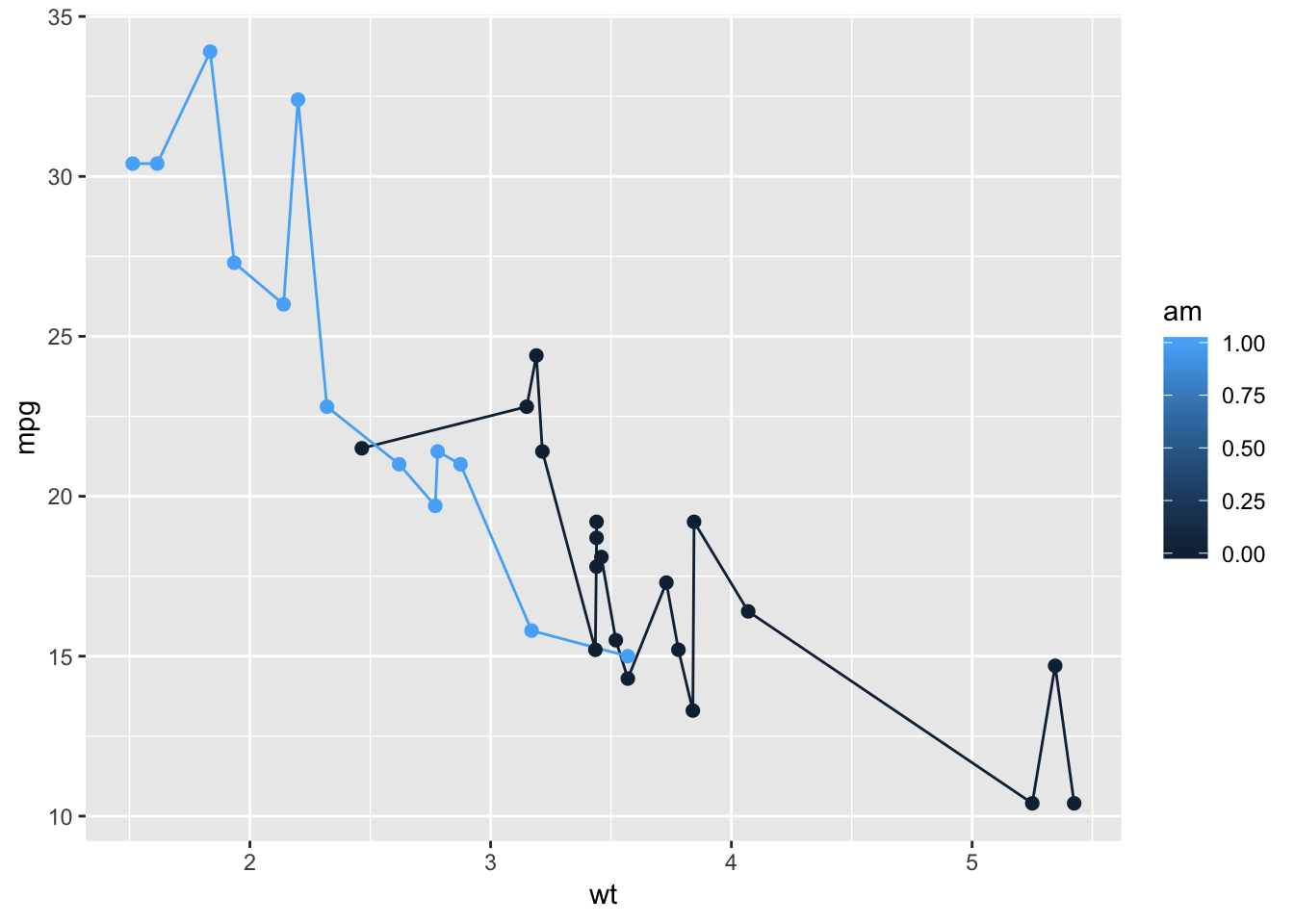``````# install.packages("ggplot2")
library(ggplot2)``````
``## Warning: package 'ggplot2' was built under R version 3.5.2``
``````# Assign built-in data "mtcars" to a new variable "mycars"
mycars <- mtcars``````

# 1. Histogram of MPG

`````` # use the mpg in "mycars" dataframe as X variable to create a histogram
# set the bin width to be 2, border color to be white, and bin color to be black
ggplot(mycars, aes(x=mpg)) + geom_histogram(binwidth = 2, color="white", fill="black")``````# 2. Boxplots of mpg by cyl

`````` # use data "mycars" to create one boxplot for each factor in "cyl" column in terms of their mpg value
ggplot(mycars, aes(x=factor(cyl), y=mpg)) + geom_boxplot() +
# add lable "cyl" to x-axis and lable "mpg" to y-axis
xlab('cyl') + ylab('mpg')``````# 3. MultiLine chart of wt on the x-axis, mpg for the y-axis

``````# group the data by "am", so one line is created for each category in "am" with different color
ggplot(mycars, aes(x=wt, y=mpg, group=am, color=am)) + geom_line() +
# add points of size 2 to the lines
geom_point(size=2)``````# 4. Barchart

``````# create a barchart with the x-axis being the name of each car, and the height being wt
# stat="identity" sets the heights of the bars equal to values in the data, instead of the number of cases in each group
ggplot(mycars, aes(x=rownames(mycars), y=wt)) + geom_bar(stat="identity") +
# rotate the x-axis lables for 45 degrees
# hjust = 0 means left-justified labels, 1 means right-justified labels
theme(axis.text.x=element_text(angle=45, hjust=1))``````